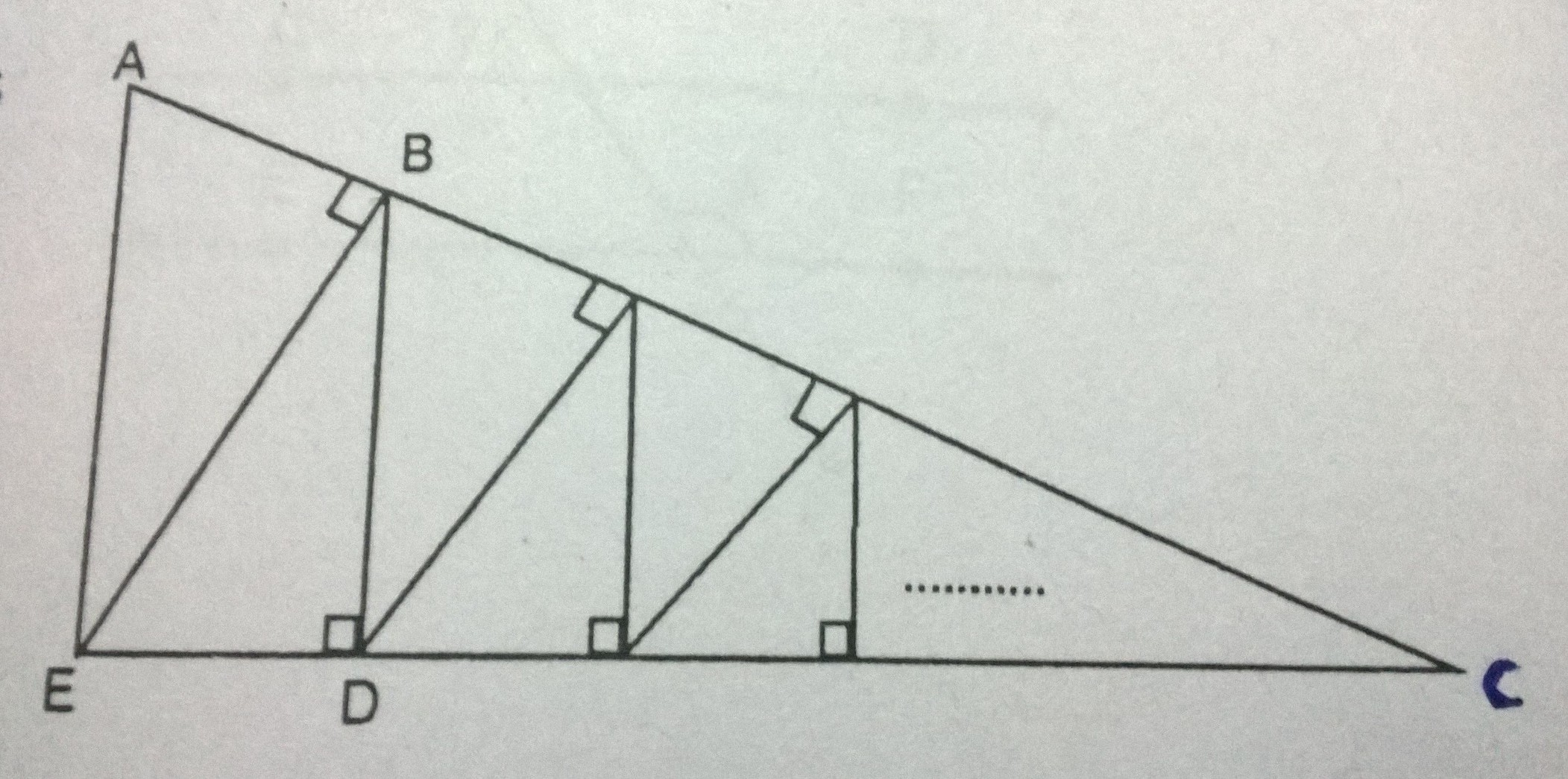# Zig Zag Zig Zag

Geometry Level 3An infinite series of similar triangles converge to a point C . If $\overline{AE} = 16 , \overline{ED} = 8$ . Denote $x$ as the sum of all the vertical segments: $\overline{AE} + \overline{BD} + \ldots$

Find the value of $x$.

×